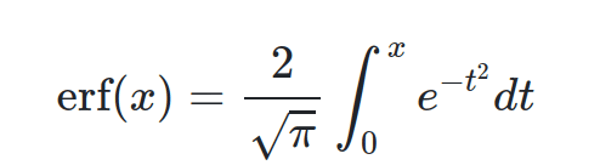Trusted answers to developer questions
Trusted Answers to Developer Questions

Related Tags

golang

# What is the Erf function in Golang?

Faraz Karim

Grokking Modern System Design Interview for Engineers & Managers

Ace your System Design Interview and take your career to the next level. Learn to handle the design of applications like Netflix, Quora, Facebook, Uber, and many more in a 45-min interview. Learn the RESHADED framework for architecting web-scale applications by determining requirements, constraints, and assumptions before diving into a step-by-step design process.

## The Erf function

The Go programming language uses the Erf function to find the error function of a certain decimal number.

To use this function, you must import the math package in your file and access the Erf function within it using the . notation (math.Erf). Here, Erf is the actual function, while math is the Go package that stores the definition of this function.

## The error function

The error function (known as the Gauss error function) is a sigmoid function used in statistics, partial differential equations to describe diffusion, and probability. It is represented using erf(x) and defined by the following formula:## Function definition

The definition of the Erf function inside the math package is:

## Parameters

The Erf function takes a single argument of type float64. This argument represents the decimal number whose error function you want to find.

## Return value

The Erf function returns a single value of type float64. This value represents the error function for a particular input argument.

Following are two types of return values only used by the function under certain circumstances:

• NAN: Not a number or NAN is returned in all cases where the input argument is of undefined value.

• (±)1: The Erf function returns +1 if the input argument has a value equalling positive infinity and -1 for negative infinity.

Giving an empty argument or an argument that is not a numeric value results in an error.

## Examples

Following is a simple example where we find out the error function of a positive decimal value:

package mainimport ("fmt"		"math")func main() {	x := 0.8	y := math.Erf(x)	fmt.Print("The error function of ", x, "is ", y)}

The following example shows how the Erf function deals with an argument with an infinite value:

package mainimport ("fmt"		"math")func main() {	zero := 0.0	y := math.Erf(-1/zero)	fmt.Print("The error function of -Inf is ", y)}

RELATED TAGS

golang

CONTRIBUTOR

Faraz Karim